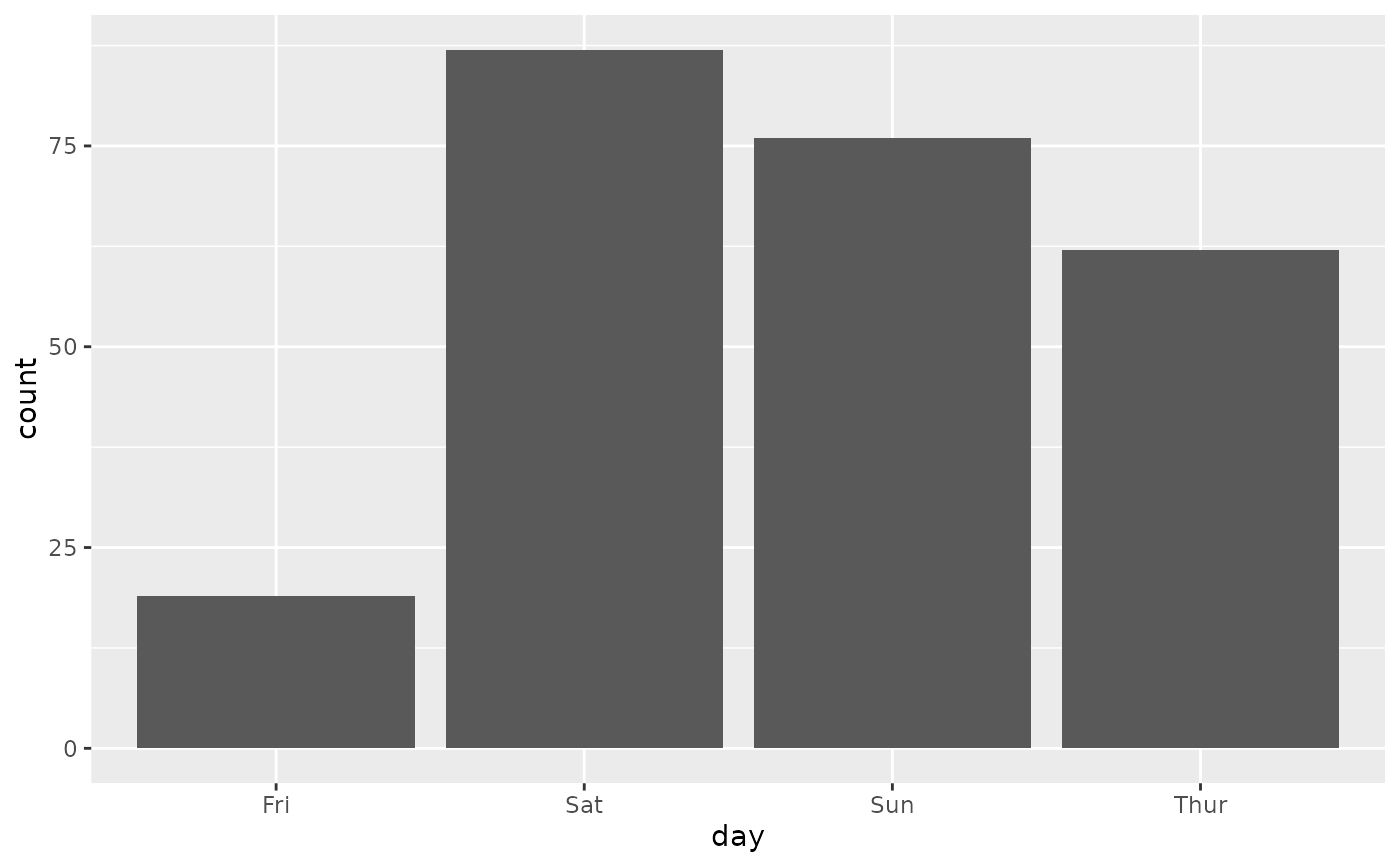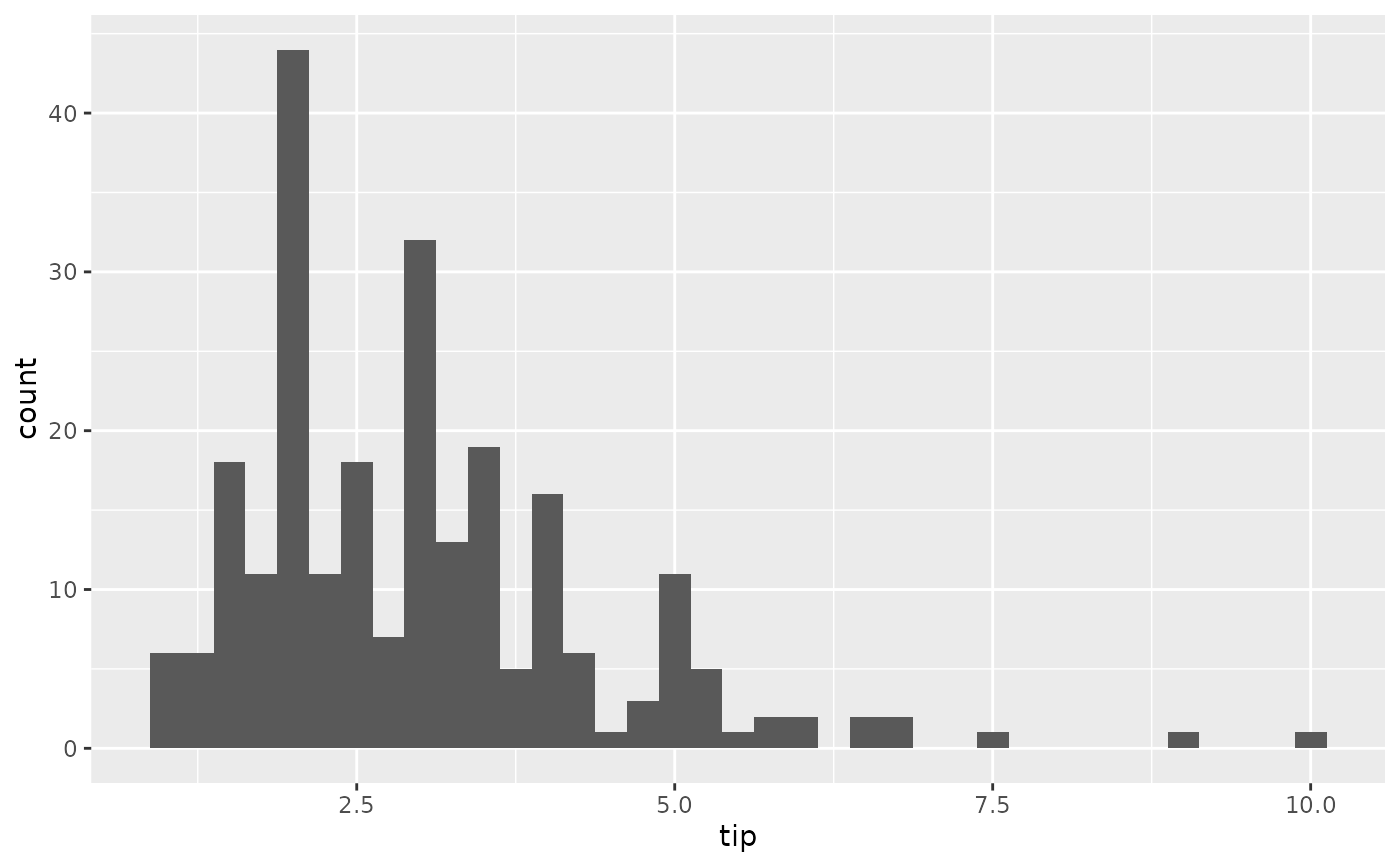Displays a bar plot for the diagonal of a ggpairs plot matrix.

ggally_barDiag(data, mapping, ..., rescale = FALSE)

## Arguments

data data set using aesthetics being used other arguments are sent to geom_bar boolean to decide whether or not to rescale the count output. Only applies to numeric data

Barret Schloerke

## Examples

# Small function to display plots only if it's interactive
p_ <- GGally::print_if_interactive

data(tips, package = "reshape")
p_(ggally_barDiag(tips, mapping = ggplot2::aes(x = day)))p_(ggally_barDiag(tips, mapping = ggplot2::aes(x = tip), binwidth = 0.25))